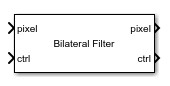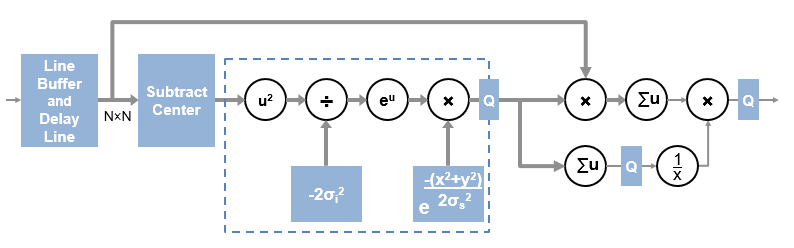Bilateral Filter

2-D bilateral filtering

• Library:
• Vision HDL Toolbox / Filtering

•Description

The Bilateral Filter block filters images while preserving edges. Some applications of bilateral filtering are denoising while preserving edges, separating texture from illumination, and cartooning to enhance edges. The filter replaces each pixel at the center of a neighborhood by an average that is calculated using spatial and intensity Gaussian filters. The block determines the filter coefficients from:

• Spatial location in the neighborhood (similar to a Gaussian blur filter)

• Intensity difference from the neighborhood center value

The block provides two standard deviation parameters for independent control of the spatial and intensity coefficients.

Ports

This block uses a streaming pixel interface with a pixelcontrol bus for frame control signals. This interface enables the block to operate independently of image size and format. All Vision HDL Toolbox™ blocks use the same streaming interface. The block accepts and returns a scalar pixel value and a bus that contains five control signals. The control signals indicate the validity of each pixel and its location in the frame. To convert a frame (pixel matrix) into a serial pixel stream and control signals, use the Frame To Pixels block. For a full description of the interface, see Streaming Pixel Interface.

Input

expand all

Single image pixel of a pixel stream, specified as a scalar value representing intensity. This value is interpreted on the range [0,1], assuming the entire range of the input data type. Integer and fixed-point data types larger than 16 bits are not supported.

double and single data types are supported for simulation, but not for HDL code generation.

Data Types: uint8 | uint16 | int8 | int16 | fixed point | Boolean | double | single

The pixelcontrol bus contains five signals. The signals describe the validity of the pixel and its location in the frame. For more information, see Pixel Control Bus.

Data Types: bus

Output

expand all

Single image pixel in a pixel stream, returned as a scalar value representing intensity. Integer and fixed-point data types larger than 16 bits are not supported.

double and single data types are supported for simulation, but not for HDL code generation.

Data Types: uint8 | uint16 | int8 | int16 | fixed point | Boolean | double | single

The pixelcontrol bus contains five signals. The signals describe the validity of the pixel and its location in the frame. For more information, see Pixel Control Bus.

Data Types: bus

Parameters

expand all

Main

Size of the image region used to compute the average, specified as an N-by-N pixel square.

Spatial standard deviation target used to compute coefficients for the spatial Gaussian filter, specified as a positive real number. This parameter has no limits, but recommended values are from 0.1 to 10. At the high end, the distribution becomes flat and the coefficients are small. At the low end, the distribution peaks in the center and has small coefficients in the rest of the neighborhood. These boundary values also depend on the neighborhood size and the data type used for the coefficients.

Intensity standard deviation target used to compute coefficients for the intensity Gaussian filter, specified as a positive real number. This parameter has no limits, but recommended values are from 0.1 to 10. At the high end, the distribution becomes flat and the coefficients are small. At the low end, the distribution peaks in the center and has small coefficients in the rest of the neighborhood. These boundary values also depend on the neighborhood size and the data type used for the coefficients.

When the intensity standard deviation is large, the bilateral filter acts more like a Gaussian blur filter, because the intensity Gaussian has a lower peak. Conversely, when the intensity standard deviation is smaller, edges in the intensity are preserved or enhanced.

• Constant — Interpret pixels outside the image frame as having a constant value.

• Replicate — Repeat the value of pixels at the edge of the image.

• Symmetric — Set the value of the padding pixels to mirror the edge of the image.

• Reflection — Set the value of the padding pixels to reflect around the pixel at the edge of the image.

• None — Exclude padding logic. The block does not set the pixels outside the image frame to any particular value. This option reduces the hardware resources used by the block and the blanking required between frames but affects the accuracy of the output pixels at the edges of the frame. To maintain pixel stream timing, the output frame is the same size as the input frame. However, to avoid using pixels calculated from undefined padding values, mask off the KernelSize/2 pixels around the edge of the frame for downstream operations. For details, see Increase Throughput with Padding None.

Specify an integer to pad the boundary of the input image. The block casts this value to the same data type as the input pixel.

Dependencies

To enable this parameter, set the Padding method parameter to Constant.

Size of line memory buffer, specified as a positive integer. Choose a power of two that accommodates the number of active pixels in a horizontal line. If you specify a value that is not a power of two, the buffer uses the next largest power of two.

If you specify a value that is not a power of two, the block uses the next largest power of two.

Data Types

When the input is any integer or fixed-point data type, the algorithm uses fixed-point arithmetic for internal calculations. This option does not apply when the input data type is single or double.

When the input is any integer or fixed-point data type, the algorithm uses fixed-point arithmetic for internal calculations. By default, fixed-point values saturate on overflow. This option does not apply when the input data type is single or double.

Specify an unsigned data type that can represent values less than 1. The coefficients usually require a data type with more precision than the input data type. The block calculates the coefficients based on the neighborhood size and the values of Intensity standard deviation and Spatial standard deviation. Larger neighborhoods spread the Gaussian function such that each coefficient value is smaller. A larger standard deviation flattens the Gaussian so that the coefficients are more uniform in nature, and a smaller standard deviation produces a peaked response.

Note

If you try a data type and after quantization, more than half of the coefficients become zero, the block issues a warning. If all the coefficients are zero after quantization, the block issues an error. These messages mean that the block was unable to express the requested filter by using the data type specified. To avoid this issue, choose a higher-precision coefficient data type or adjust the standard deviation targets.

The filtered pixel values are cast to this data type.

Tips

• When you use a block with an internal line buffer inside an Enabled Subsystem (Simulink), the enable signal pattern must maintain the timing of the pixel stream, including the minimum blanking intervals. If the enable pattern corrupts the timing of the pixel stream, you might see partial output frames, corrupted pixel stream control signals, or mismatches between Simulink® and HDL simulation results. You may need to extend the blanking intervals to accommodate for cycles when the enable is low. For more information, see Configure Blanking Intervals.

Algorithms

expand all

The bilateral filter can be described as a Gaussian filter in the spatial dimension that modifies the coefficients of a second Gaussian filter that operates on intensity.

The algorithm stores N-1 lines so that it can form an N-by-N matrix of pixels matching the Neighborhood size. Then it applies two Gaussian filters on each neighborhood. The filter coefficients are calculated from the spatial and intensity standard deviations.The Subtract Center operation produces a pixel value of zero at the center of the neighborhood. For hardware implementation, and for simulation of fixed-point or integer data types, the calculation in the dashed region is implemented with a lookup table of precomputed values for each pixel. Because the center value is always zero, u2 and eu are always one and are not computed. For floating-point input, the simulation computes u2 and eu as shown. The output of the dashed region uses the coefficient data type that you specified. The Q blocks in the diagram show quantization points.

The algorithm implements the final normalization step with a reciprocal lookup table in the hardware implementation. The lookup table has 2048 locations, so the coefficient sum is quantized to the most significant 11 bits. The reciprocal values use the output data type that you specified, plus a minimum of two integer bits if the data type does not already include them. The reciprocal lookup value for a zero sum is the maximum representable value in the coefficient data type. For floating-point normalization, the simulation detects a zero sum and instead divides by eps() of the dividend.

The output pixel value is then cast to the output data type that you specified. The filter uses the entire range of the data type, so if your color space uses less than the full range, you may need to rescale the pixel values.

Note

When filtering multicomponent (color) pixels, false colors can occur, unless the operation is done in a color space based on human perception, such as CIELab. Bilateral filtering of the R'G'B' color space is not recommended.

Extended Capabilities

Objects

Introduced in R2017b

Vision HDL Toolbox DocumentationGet trial now